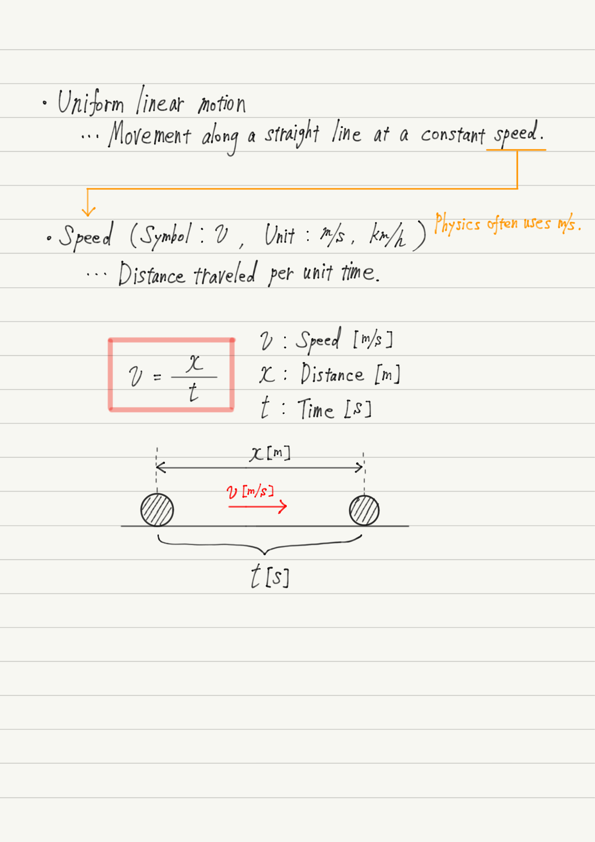# Uniform Linear Motion

The first topic of mechanics is uniform linear motion. Since this is the same content learned in junior high school, let’s review it starting with the easy parts.

## What is uniform linear motion?

Uniform linear motion is, as the name implies, “motion with the same speed and in the same direction”.

Frankly speaking, we rarely see them in our daily lives. It is too convenient that neither the direction nor the speed of the wind stays the same all the time.
What is important in physics, however, is to first think in terms of the simplest model before dealing with complex phenomena. In other words, uniform linear motion is the foundation of all the motions we will learn from now on!

Let us now summarize the “speed” of motion.

## Summary of this lectureThe important part of the definition of speed is that it is based on time.
Usually, when competing for speed, the distance is determined first and then the time is measured, as in track and field events. The person who runs the specified distance in the shortest time is the fastest.
In physics, however, the time is determined first and then the distance is measured. The person who runs the longest distance within the specified time is the fastest.
If you do not understand the difference between these definitions, you will stumble in your calculations.

## Next Time

In the next lecture, we will learn about graphs of uniform linear motion.

https://www.yukimura-physics.com/entry/dyn-f02

error: Content is protected !!